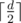CF746F Music in Car

• 21通过
• 46提交
• 题目来源
• 评测方式 RemoteJudge
• 标签
• 难度 省选/NOI-
• 时空限制 1000ms / 256MB
• 提示：收藏到任务计划后，可在首页查看。

推荐的相关题目 显示

本题征求翻译。如果你能提供翻译或者题意简述，请 提交翻译 ，感谢你的贡献。

题目描述

Sasha reaches the work by car. It takes exactly $k$ minutes. On his way he listens to music. All songs in his playlist go one by one, after listening to the $i$ -th song Sasha gets a pleasure which equals $a_{i}$ . The $i$ -th song lasts for $t_{i}$ minutes.

Before the beginning of his way Sasha turns on some song $x$ and then he listens to the songs one by one: at first, the song $x$ , then the song $(x+1)$ , then the song number $(x+2)$ , and so on. He listens to songs until he reaches the work or until he listens to the last song in his playlist.

Sasha can listen to each song to the end or partly.

In the second case he listens to the song for integer number of minutes, at least half of the song's length. Formally, if the length of the song equals $d$ minutes, Sasha listens to it for no less thanminutes, then he immediately switches it to the next song (if there is such). For example, if the length of the song which Sasha wants to partly listen to, equals $5$ minutes, then he should listen to it for at least $3$ minutes, if the length of the song equals $8$ minutes, then he should listen to it for at least $4$ minutes.

It takes no time to switch a song.

Sasha wants to listen partly no more than $w$ songs. If the last listened song plays for less than half of its length, then Sasha doesn't get pleasure from it and that song is not included to the list of partly listened songs. It is not allowed to skip songs. A pleasure from a song does not depend on the listening mode, for the $i$ -th song this value equals $a_{i}$ .

Help Sasha to choose such $x$ and no more than $w$ songs for partial listening to get the maximum pleasure. Write a program to find the maximum pleasure Sasha can get from the listening to the songs on his way to the work.

输入输出格式

输入格式：

The first line contains three integers $n$ , $w$ and $k$ ( $1<=w<=n<=2·10^{5}$ , $1<=k<=2·10^{9}$ ) — the number of songs in the playlist, the number of songs Sasha can listen to partly and time in minutes which Sasha needs to reach work.

The second line contains $n$ positive integers $a_{1},a_{2},...,a_{n}$ ( $1<=a_{i}<=10^{4}$ ), where $a_{i}$ equals the pleasure Sasha gets after listening to the $i$ -th song.

The third line contains $n$ positive integers $t_{1},t_{2},...,t_{n}$ ( $2<=t_{i}<=10^{4}$ ), where $t_{i}$ equals the length of the $i$ -th song in minutes.

输出格式：

Print the maximum pleasure Sasha can get after listening to the songs on the way to work.

输入输出样例

输入样例#1： 复制
7 2 11
3 4 3 5 1 4 6
7 7 3 6 5 3 9

输出样例#1： 复制
12

输入样例#2： 复制
8 4 20
5 6 4 3 7 5 4 1
10 12 5 12 14 8 5 8

输出样例#2： 复制
19

输入样例#3： 复制
1 1 5
6
9

输出样例#3： 复制
6

输入样例#4： 复制
1 1 3
4
7

输出样例#4： 复制
0


说明

In the first example Sasha needs to start listening from the song number $2$ . He should listen to it partly (for $4$ minutes), then listen to the song number $3$ to the end (for $3$ minutes) and then partly listen to the song number $4$ (for $3$ minutes). After listening to these songs Sasha will get pleasure which equals $4+3+5=12$ . Sasha will not have time to listen to the song number $5$ because he will spend $4+3+3=10$ minutes listening to songs number $2$ , $3$ and $4$ and only $1$ minute is left after that.

提示
标程仅供做题后或实在无思路时参考。
请自觉、自律地使用该功能并请对自己的学习负责。
如果发现恶意抄袭标程，将按照I类违反进行处理。